# What is unordered_map in C++

## Introduction to unordered_map in C++

C++ unordered_map containers are faster than typical map containers. This is attributed to the unordered map being implemented using hash tables.

### Definition and purpose

C++ unordered_map is a  built-in container that stores elements in key-value pairs. The values in unordered_map containers are not internally defined in any specific fashion.

The data types of key and mapped values can either be predefined or executed at the time, and values are inserted into the container.

Internally C++ unordered_map is implemented using Hash Table; the key provided to map is hashed into indices of a hash table which is why the performance of data structure depends on the hash function a lot, but on average, the cost of search, insert, and delete from the hash table is O(1).

The key values of the map are linked to the hash values of the table, which are then organized into separate buckets. This way, once the hash values are calculated, the compiler can quickly access the exact bucket where the specified element is located.

### Why should you use unordered_map?

An unordered_map is very fast and can store elements as key-value pairs in non-sorted order. The time complexity for operations in unordered_map is O(1).

### When should you use unordered_map?

You should use the unordered_map in C++ when you need to keep count of some data, and no order is required. You can also use it when you only want to access a single element.

## Creating and Initializing unordered_map

### Syntax

``unordered_map<key_datatype, mapped_datatype> map_name;``

### Example

``````#include <iostream>
#include <iterator>
#include <unordered_map>
using namespace std;
int main()
{
unordered_map<int, char> map1;
unordered_map<int, char>::iterator cursor;
map1 = 'a';
map1 = 'b';
cout << "KEY\tELEMENT" << endl;
for (cursor = map1.begin(); cursor != map1.end(); cursor++)
{
cout << cursor->first;
cout << '\t' << cursor->second << '\n'
<< endl;
}
}``````

Output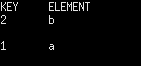See the above code is a simple program in C++ that demonstrates using an unordered_map container.

1. Includes the necessary headers, `iostream`, `iterator`, and `unordered_map`, from the C++ Standard Library.

2. Declares an unordered_map container named `map1` that maps integers to characters.
3. Declares an iterator named `cursor` to iterate through the elements of `map1`.
4. Inserts elements into the `map1` container using the array operator `[]`. The elements are key-value pairs of integers and characters, `1` maps to `'a'` and `2` maps to `'b'`.
5. Prints a header “KEY\tELEMENT” and iterates through the elements of the `map1` container using a for loop. The loop starts at the beginning of the `map1` container using `map1.begin()` and continues until the end of the container, as indicated by `map1.end()`.
6. Within the for loop, the key and value of each element are printed using the arrow operator `->` and `first` and `second` member functions, respectively.
7. The end result will be a table with two columns “KEY” and “ELEMENT”, where each row corresponds to a key-value pair in the `map1` container.

## Comparison of C++ Unordered_maps

### With usual map containers

In map containers, elements are placed in proper order, whereas in unordered maps, the order is entirely random. This is attributed to map containers implemented using tree structures with specific traversal paths. Still, the unordered maps are done using the hash tables.

But this makes the unordered maps quite faster and accessible with time complexity of O(1), compared to typical map containers having O(nlogn).

### With unordered sets

In unordered sets, the elements are not stored in key-value pairs but rather just in the form of keys used to judge the presence of items in a set.

But with unordered maps, we can have the frequencies of the presence of an element except for just its presence.

## Properties and Functions of unordered_map

### begin()

The begin() function returns a bidirectional iterator that points to the very first element of the map. This function demands no parameter to be passed and shows an error when it is done.

#### Syntax:

`mapcontainer_name.begin();`

See the following program.

```#include <iostream>
#include <iterator>
#include <map>
using namespace std;
int main()
{
map<int, char> map1;
map<int, char>::iterator cursor;
map1 = 'a';
map1 = 'b';
map1.begin();
}```

(Returns an iterator pointing to the first element of the map.)

### end()

The end() function is meant to return a bidirectional iterator pointing to the element next to the last element in the map container.

Just like begin() function, this, too, doesn’t require any parameter. Also, since it points to a non-valid element, it cannot be dereferenced.

#### Syntax:

`mapcontainer_name.end();`

See the following program.

```#include <iostream>
#include <iterator>
#include <map>
using namespace std;
int main()
{
map<int, char> map1;
map<int, char>::iterator cursor;
map1 = 'a';
map1 = 'b';
map1.end();
}
```

(Returns an iterator pointing to the next of the last element of the map.)

### at()

The at() function is used to access the mapped values in the unordered map container whose key values you know.

#### Syntax:

`mapcontainer_name.at(key_value);`

See the following program.

```#include <iostream>
#include <iterator>
#include <unordered_map>
using namespace std;
int main()
{
unordered_map<int, char> map1;
unordered_map<int, char>::iterator cursor;
map1 = 'a';
map1 = 'b';
cout << map1.at(2) << endl;
}
```

See the following output.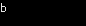### bucket()

The bucket() function is used to get the bucket number, which holds the mapped value of the key value you provide as a parameter.

#### Syntax:

`mapcontainer_name.bucket(key_value);`

See the following program.

```#include <iostream>
#include <iterator>
#include <unordered_map>
using namespace std;
int main()
{
unordered_map<int, char> map1;
unordered_map<int, char>::iterator cursor;
map1 = 'a';
map1 = 'b';
cout << map1.bucket(2) << endl;
}
```

See the following output.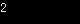### bucket_count()

The bucket_count() function is used to get the total number of buckets in an unordered map container. This function doesn’t require any parameters.

#### Syntax:

`mapcontainer_name.bucket(key_value);`

See the following program.

```#include <iostream>
#include <iterator>
#include <unordered_map>
using namespace std;
int main()
{
unordered_map<int, char> map1;
unordered_map<int, char>::iterator cursor;
map1 = 'a';
map1 = 'b';
cout << map1.bucket_count() << endl;
}
```

See the following output.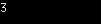### bucket_size()

The bucket_size() function is used to get the number of elements in a single bucket of the unordered map container.

#### Syntax

`mapcontainer_name.bucket_size(bucket_value);`

See the following program.

```#include <iostream>
#include <iterator>
#include <unordered_map>
using namespace std;
int main()
{
unordered_map<int, char> map1;
unordered_map<int, char>::iterator cursor;
map1 = 'a';
map1 = 'b';
cout << map1.bucket_size(2) << endl;
}
```

See the following output.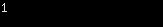### count()

The count() function gets several elements with the same key value in a container. But since a map container allows only one mapped value with a certain key value, this function only checks whether or not a key is present.

#### Syntax:

`mapcontainer_name.count(key_value);`

See the following program.

```#include <iostream>
#include <iterator>
#include <unordered_map>
using namespace std;
int main()
{
unordered_map<int, char> map1;
unordered_map<int, char>::iterator cursor;
map1 = 'a';
map1 = 'b';
cout << map1.count(1) << endl;
}
```

See the following output.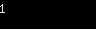### equal_range()

The equal_range() function returns the initial and ends iterators of the range K, which contains all the elements whose key is equal to K.

Now, since, in map containers, there are no duplicate keys, the range contains at most one value.

#### Syntax:

`mapcontainer_name.equal_range(key_value);`

See the following program.

```#include <iostream>
#include <iterator>
#include <unordered_map>
using namespace std;
int main()
{
unordered_map<int, char> map1;
unordered_map<int, char>::iterator cursor;
map1 = 'a';
map1 = 'b';
auto len = map1.equal_range(1);
for (auto m = len.first; m != len.second; m++)
{
cout << "Key : " << m->first << endl;
cout << "Value : " << m->second << endl;
}
}
```

See the following output.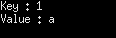That’s it for this tutorial.

Categories C++

This site uses Akismet to reduce spam. Learn how your comment data is processed.